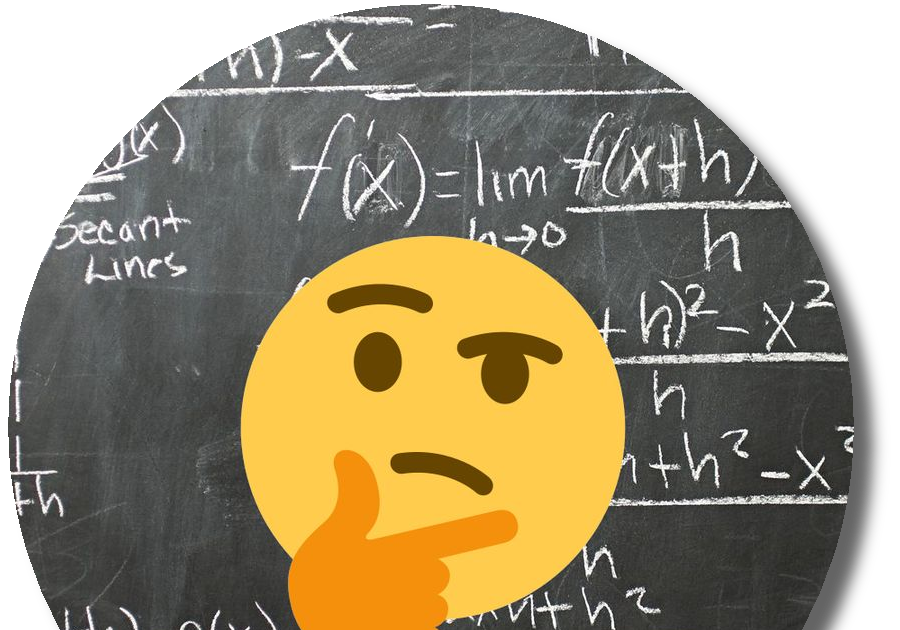NPR Sunday Puzzle (Feb 26, 2023): It Doesn’t Add Up

Q: Take this equation: 14 + 116 + 68 = 47. Clearly this doesn’t work mathematically. But it does work in a nonmathematical way. Please explain.

It makes about as much sense as 22 + 11 = 26 + 39.

Edit: My equation turns into Ti + Na = Fe + Y, referring back to the recent chemical elements puzzle where Tina Fey was the answer.

A: Looking at the periodic table and replacing the numbers with the chemical symbols, we get Si + Lv + Er = Ag